﻿ Vibration of Non-Homogeneous Rectangular Plate Having Parabolically Varying Thickness in Both Directions with Exponentially Temperature Distribution

### Vibration of Non-Homogeneous Rectangular Plate Having Parabolically Varying Thickness in Both Direct...

Arun Kumar Gupta, Vaibhav PanwarOPEN ACCESSPEER-REVIEWED

## Vibration of Non-Homogeneous Rectangular Plate Having Parabolically Varying Thickness in Both Directions with Exponentially Temperature Distribution

Arun Kumar Gupta1,, Vaibhav Panwar2

1Department of Mathematics, M. S. College, Saharanpur, U.P., India

2Department of MCA, Institute of Management Studies, Dehradun, India

### Abstract

An analysis is presented for frequencies of non-homogeneous rectangular plates of bi-parabolically thickness variation with exponentially temperature distribution on the basis of classical plate theory. An approximate but quiet convenient frequency equation is derived by using Rayleigh-Ritz technique with a two term deflection function. Effect of non-homogeneity together with taper constants and thermal gradient on the natural frequencies of vibration of a clamped rectangular plate on the first two modes of vibration have been analysed. Results are presented in tabular and graphical form both.

### At a glance: Figures

123
Prev Next

• Gupta, Arun Kumar, and Vaibhav Panwar. "Vibration of Non-Homogeneous Rectangular Plate Having Parabolically Varying Thickness in Both Directions with Exponentially Temperature Distribution." Applied Mathematics and Physics 1.4 (2013): 94-102.
• Gupta, A. K. , & Panwar, V. (2013). Vibration of Non-Homogeneous Rectangular Plate Having Parabolically Varying Thickness in Both Directions with Exponentially Temperature Distribution. Applied Mathematics and Physics, 1(4), 94-102.
• Gupta, Arun Kumar, and Vaibhav Panwar. "Vibration of Non-Homogeneous Rectangular Plate Having Parabolically Varying Thickness in Both Directions with Exponentially Temperature Distribution." Applied Mathematics and Physics 1, no. 4 (2013): 94-102.

 Import into BibTeX Import into EndNote Import into RefMan Import into RefWorks

### 1. Introduction

Vibration phenomenon, common in mechanical devices and structures, is undesirable in many cases, such as machine tools. But this phenomenon is not always unwanted; for example, vibration is needed in the operation of vibration screens. Over time, engineers have become increasingly conscious of the importance of the elastic behavior of plates as well as the natural frequencies and mode shapes of the plates, which, from a technical point of view, is indispensable information. For reasons of both practical and academic interests, numerous publications concerned with the vibration of plates have been published. Vibration problems of elastic plate are very much comprehensive due to various geometrical shapes with complications of anisotropy, visco-elastic, non-homogeneity, variable thickness, surrounding media, in plane force, large deflections, elastic foundation, shear deformation and rotatory inertia, simple and mixed boundary conditions etc. The survey of literature on the subject shows the fact that relatively less work has been done on vibration problems of non-homogeneous elastic plate having variable thickness in both directions with thermal gradient.

Tapered and non-homogeneous plates are used quite often to model structures which have a wide variety of applications.

Various numerical techniques have been employed to study the vibration characteristics of plates of various geometries by Leissa . The plate type structural components in aircraft and rockets have to operate under elevated temperatures which cause non-homogeneity in the plate material i.e. elastic constants of the material become functions of the space variables. In an up-to-date survey of literature, authors have come across various models to account for non-homogeneity of plate material proposed by researchers dealing with vibration. Leissa  has given the solution for rectangular plate of variable thickness.

Laura and Gutierrez  discussed vibration analysis of a rectangular plate subjected to a thermal gradient. Singh and Saxena  analyzed the transverse vibration of rectangular plate with bi-directional thickness variation. Gupta et al.  studied the thermal gradient effect on vibration of a non-homogeneous orthotropic rectangular plate having bi-direction linearly thickness variation. Gupta et al.  observed the thermal effect on vibration of non-homogeneous orthotropic rectangular plate having bi-directional parabolically varying thickness. Tomar and Gupta [7, 8] studied the effect of thermal gradient on frequencies of orthotropic rectangular plate of variable thickness in one and two direction. Tomar and Gupta  have been discussed the vibration problem of orthotropic rectangular plate of variable thickness having exponentially temperature variation. Raju  studied the vibration of thin elastic plates of linearly variable thickness. Gupta et al.  studied the thermal gradient effect on vibrations of non-homogeneous rectangular plate having continuously parabolically varying thickness in both directions. Chakarverty  discussed the vibration of plates. Li  studied the vibration analysis of rectangular plate with general elastic boundary supports.

The object of the present study is to determine the effect of exponentially temperature on free vibration of a clamped non- homogeneous rectangular plate having bi-directions parabolically thickness variations. In order to evaluate frequencies for first two modes of vibration, Rayleigh Ritz method is applied. The non-homogeneity is assumed, to arise due to the variation in the density of the plate material, linear along the length of the plate. The frequencies for first two modes of vibration are calculated for clamped plate, for different values of non-homogeneity constant, two taper constants and temperature gradient.

### 2. Method of Analysis

Let us assume that steady one dimensional exponentially temperature distribution T along x- axis as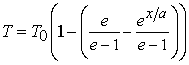(1)

where T is the temperature excess above the reference temperature at a distance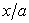and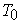is the temperature excess above the reference temperature at the end of the plate i.e. at x=a.

Temperature dependence of the modulus of elasticity for most of engineering materials can be expressed in the form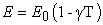(2)

where E0 is the value of the Young's modulus at reference temperature i.e. T=0and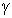is the slope of the variation of E with T. Thus modulus variation become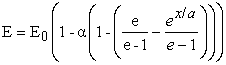(3)

where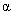= γ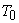(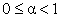), a parameter known as thermal gradient.

Assuming that thickness varies parabolically in both directions and it is of the form as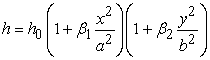(4)

where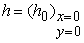and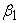&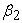are taper constants in x- and y- directions ,respectively.

Let us assume that density varies linearly in x-direction as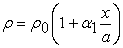(5)

where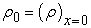and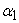is a non-homogeneity constant.

Deflection function for free transverse vibrations of the plate can be written as, in the form of Levy type solution,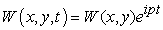(6)

Strain energy V, when the plate is executing transverse vibration of mode shape W (x, y), :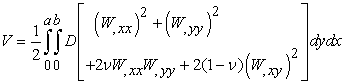(7)

and Kinetic energy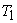is written as,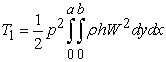(8)

The flexural rigidity of the plate can now be written as (assuming Poisson’s ratio ν is constant):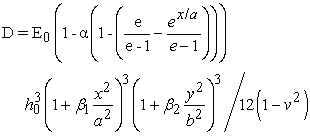(9)

Using equations (4) and (5) in equation (8), one has,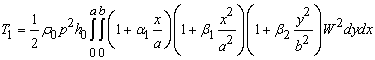(10)

Rayleigh Ritz technique requires that the maximum strain energy and maximum kinetic energy must be equal i.e.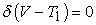(11)

The boundary conditions are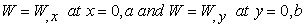(12)

Taking two term deflection function as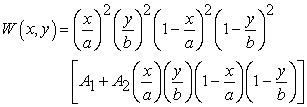(13)

where A1 and A2 are constants.

Using equation (9) in equation (7), one get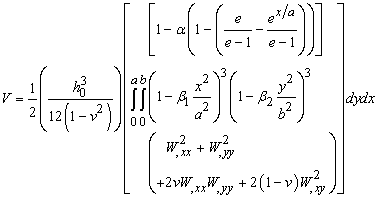(14)

Substituting equations (10) and (14) in equation (11), one has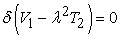(15)

where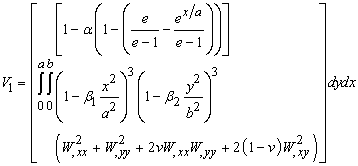(16)

and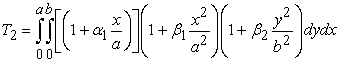(17)

and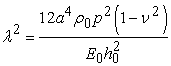(18)

is a frequency parameter.

The two unknown parameters A1 and A2 , containing in equation (15) can be evaluated as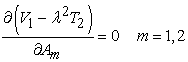(19)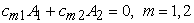(20)

where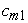and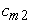(m=1, 2) involves parametric constants and frequency parameter.

For a non- zero solution, determinant of coefficient of equation (20) must be zero.

In this way the frequency equation comes out to be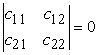(21)

A quadratic equation in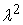can be obtain from equation (21), which gives the two values of.

### 3. Results and Discussion

The frequency equation (21) provides values of the frequency parameter for various values of plate parameters. In the present investigation, vibration behavior of a clamped non-homogeneous plate with bi-parabolic thickness variation and exponentially temperature effect is considered for different values of two taper constants, thermal gradient and non-homogeneity constant with constant aspect ratio 1.5. The frequency equation (21), being quadratic in, will give two roots that correspond to the first and second modes of vibration, respectively. Poisson’s ratio is taken as 0.3. These results are presented in Tables 1-8 and explain by Figures 1 through 8.

The authenticities of the results are confirmed by comparing them with those of Leissa  for unheated uniform homogeneous rectangular plate and with the authors published results  for unheated, non-uniform and non-homogeneous rectangular plate.

From Table 1, it can be concluded that with the increase in thermal gradient, the frequency decreases for both mode of vibration. Figure has been draw for the following two cases:-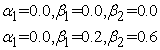It is to be clear from the figure that frequency parameter decreases sharply for second mode of vibration as compared to the first mode of vibration.

#### Table 2. Values of frequency parameter ‘λ’ for different values of non-homogeneity parameter ‘α1’ and constant aspect ratio a/b = 1.5

Table 2 shows the variation of non-homogeneity constant with frequency parameter, for both modes of vibration. The figure has been plotted for the following two cases:-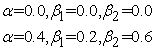From figure it is observed that for both modes of vibration, the frequency decreases with increase in non-homogeneity for both heated and unheated plate.

#### Table 5. Values of frequency parameter ‘λ’ for different values of taper constant ‘β1’ and constant aspect ratio a/b = 1.5

Table 3, Table 4 and Table 5 show the variation of frequency parameter with taper constantfor various values of thermal gradient, non-homogeneity constant and taper constantfor a clamped rectangular plate for the following cases: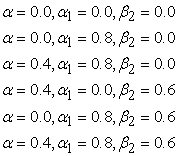It is observed from the figures that for both modes of vibration, frequency parameter increases with increases in taper constantfor heated or unheated and homogeneous or non-homogeneous plate.

#### Table 8. Values of frequency parameter ‘λ’ for different values of taper constant ‘β2’ and constant aspect ratio a/b = 1.5

Table 6, Table 7 and Table 8 contain data of the frequency parameter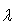with the taper constantfor the first and second mode, respectively, for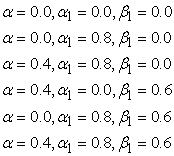It is observed from figures that for both modes of vibration, frequency parameter increases with increases in taper constantfor heated or unheated and homogeneous or non-homogeneous plate.

Further it is interesting to note that effect of taper in x-direction for non-homogeneity is more dominate in comparison in y-direction.

### 4. Conclusions

The present work shows effect of exponentially temperature on the frequencies of non- homogeneous clamped rectangular plate of variable thickness with two term deflection. Thickness varies parabolically in both directions and density varies linearly in x- direction. Rayleigh-Ritz method is used to obtain frequencies for first two modes of vibration. The temperature effect with other plate parameters such as taper constants and non-homogeneity constant have also been considered.

It is observed that frequency parameter decreases with increasing value of non-homogeneity constant, it also decreases with increasing values of thermal gradient. The behavior of frequency parameter is found to increase with increasing values of taper constant for both the modes of vibration.

Figure 1. Variation of frequency parameter with thermal gradient α for an non-homogenous rectangular plate having Parabolically varying thick ness in both directions
Figure 2. Variation of frequency parameter with non-homogeneity parameter α1 for an non-homogenous rectangular plate having Parabolically varying thickness in both directions
Figure 3. Variation of frequency parameter with Taper Constant β1 for an non-homogenous rectangular plate having Parabolically varying thickness in both directions
Figure 4. Variation of frequency parameter with Taper Constant β1 for an non-homogenous rectangular plate having Parabolically varying thickness in both directions
Figure 5. Variation of frequency parameter with Taper Constant β1 for an non-homogenous rectangular plate having Parabolically varying thickness in both directions
Figure 6. Variation of frequency parameter with Taper Constant β2 for an non-homogenous rectangular plate having Parabolically varying thickness in both directions
Figure 7. Variation of frequency parameter with Taper Constant β2 for an non-homogenous rectangular plate having Parabolically varying thickness in both directions
Figure 8. Variation of frequency parameter with Taper Constant β2 for an non-homogenous rectangular plate having Parabolically varying thickness in both directions

### References

  Leissa A.W. (1969) Vibration of Plates NASA-SP-60.In article  Leissa A.W. (1987), Recent studies in plate vibration 1981-1985 part II, complicating effects, Shock and Vibration Dig., 19, 10-24.In article CrossRef  Laura P.A.A. and Gutierrez R.H. (1980), Vibration analysis on a rectangular plate subjected to a thermal gradient, J. Sound and Vibration, 72, 263.In article CrossRef  Singh B. and Saxena V.(1996), Transverse vibration of rectangular plate with bi-directional thickness variation, J. Sound and Vibration ,198, 51-65.In article CrossRef  Gupta A.K., Johri T. and Vats R.P. (2010) Study of thermal gradient effect on vibration of a non-homogeneous orthotropic rectangular plate having bi-direction linearly thickness variations, Meccanica, 45(3), 393-400.In article CrossRef  Gupta A.K., Johri T. and Vats R.P. (2007) Thermal effect on vibration of non-homogeneous orthotropic rectangular plate having bi-directional parabolically varying thickness, Proceeding of International Conference in World Congress on Engineering and Computer Science 2007 , San Francisco(USA), 24-26 October, 784-787.In article PubMed  Tomar J.S. and Gupta A.K. (1983) Thermal effect of frequencies of an orthotropic rectangular plate of linearly varying thickness, J. Sound and Vibration, 90(3), 325-331.In article CrossRef  Tomar J.S. and Gupta A.K. (1985) Effect of thermal gradient on frequencies of an orthotropic rectangular plate whose thickness varies in two-directions, J. Sound and Vibration, 98(2), 257-262.In article CrossRef  Tomar J.S. and Gupta A.K. (1986) Effect of exponential temperature variation on frequencies of an orthotropic rectangular plate of exponentially varying thickness, Proc. of the Workshop on ‘Computer Application in Continuum Mechanics’ (March 11 to 13), 183-188,Department of Mathematics, University of Roorkee, Roorkee .In article  Raju B.B.(1966) Vibration of thin elastic plates of linearly variable thickness, International Journal of Mechanical Sciences , 8,89-100.In article CrossRef  Gupta A.K., Panwar V. and Vats R.P. (2011) Thermal gradient effect on vibrations of non-homogeneous rectangular plate having continuously parabolically varying thickness in both directions, Journal of Experimental & Applied Mechanics, 2(1), 38-46.In article  Chakarverty S. (2009) Vibration of plate, 10, Taylor and Francis.In article  Li W.L. (2004) Vibration analysis of rectangular plate with general elastic boundary supports, J. Sound and Vibration, 273(3), 619-635.In article CrossRef  Olsson U.(1972) On free vibrations at temperature dependent material properties and transient temperature fields, J. Appl. Mach., Trans. ASME, 39, 723-726.In article CrossRef  Nowacki W. (1962) Thermoelasicity, Pergamon Press, New York.In article